# Texas Go Math Grade 8 Lesson 9.3 Answer Key Volume of Spheres

Refer to our Texas Go Math Grade 8 Answer Key Pdf to score good marks in the exams. Test yourself by practicing the problems from Texas Go Math Grade 8 Lesson 9.3 Answer Key Volume of Spheres.

## Texas Go Math Grade 8 Lesson 9.3 Answer Key Volume of Spheres

Modeling the Volume of a Sphere

A sphere is a three-dimensional figure with all points the same distance from the center. The radius of a sphere is the distance from the center to any point on the sphere.

You have seen that a cone fills $$\frac{1}{3}$$ of a cylinder of the same radius and height h. If you were to do a similar experiment with a sphere of the same radius, you would find that a sphere fills $$\frac{2}{3}$$ of the cylinder. The cylinder’s height is equal to twice the radius of the sphere.Reflect

Analyze Relationships A cone has a radius of r and a height of 2r. A sphere has a radius of r. Compare the volume of the sphere and cone.
The volume of the cone with a radius of r and a height of 2r is
Vcone = $$\frac{1}{3}$$πr2h
Vcone = $$\frac{1}{3}$$ ∙ π ∙ r2 ∙ (2r)
Vcone = $$\frac{2}{3}$$ ∙ π ∙ r3
The volume of the sphere with a radius of r is
Vsphere = $$\frac{4}{3}$$πr3
As we can see, the volume of the sphere is twice the volume of the cone.

Example 1

Find the volume of each sphere. Round your answers to the nearest tenth if necessary. Use 3.14 for π.Find the volume of each sphere. Round your answers to the nearest tenth. Use 3.14 for π.

Question 2.
A sphere has a radius of 10 centimeters. __________________
It is given that the radius of the sphere is 10 centimeters. Therefore, the volume of the sphere is:
Vsphere = $$\frac{4}{3}$$πr3
Vsphere = $$\frac{4}{3}$$ ∙ (3.14) ∙ (10)3
Vsphere ≈ 4186.7 cm3

Question 3.
A sphere has a diameter of 3.4 meters. __________________
It is given that the diameter of the sphere is 3.4 meters, which means that the radius is 1.7 meters. Therefore, the volume of the sphere is:
Vsphere = $$\frac{4}{3}$$πr3
Vsphere = $$\frac{4}{3}$$ ∙ (3.14) ∙ (1.7)3
Vsphere ≈ 20.6 m3

Example 2

Soccer balls come in several different sizes. One soccer ball has a diameter of 22 centimeters. What is the volume of this soccer ball? Round your answer to the nearest tenth. Use 3.14 for π.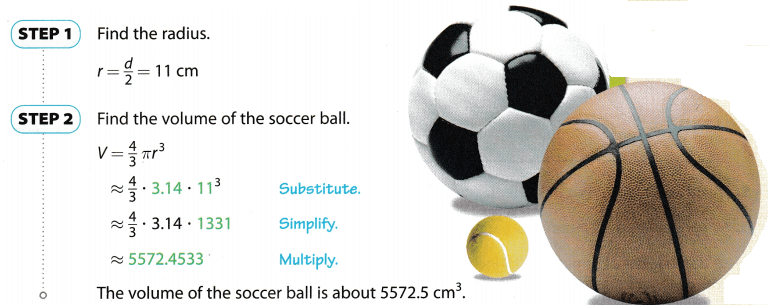Reflect

Question 4.
What is the volume of the soccer ball in terms of π, to the nearest whole number multiple? Explain your answer.
Vsphere = $$\frac{4}{3}$$πr3
Vsphere = $$\frac{4}{3}$$ ∙ π ∙ (11)3
Vsphere = $$\frac{4}{3}$$ ∙ π ∙ 1331
Vsphere = $$\frac{5324}{3}$$ ∙ π
Vsphere ≈ 1775 π
The volume of the soccer ball in terms of π, to the nearest whole number multiple, is 1775 πcm3.

Texas Go Math Grade 8 Pdf Lesson 9.3 Volume of Spheres Question 5.
Analyze Relationships The diameter of a basketball is about 1.1 times that of a soccer ball. The diameter of a tennis ball is about 0.3 times that of a soccer ball. How do the volumes of these balls compare to that of a soccer ball? Explain.
The volume of the soccer ball is
Vsoccer = $$\frac{4}{3}$$πr3
Since the diameter of a basketball is about 1.1 times that of a soccer ball, its radius is also 1.1 times that of a soccer ball. Therefore, the volume of the basketball is
Vbasketball = $$\frac{4}{3}$$ ∙ π ∙ (1.1 r)3
Vbasketball = $$\frac{4}{3}$$ ∙ π ∙ 1.331 ∙ r3
Since the diameter of a tennis ball is about 0.3 times that of a soccer ball, its radius is also 0.3 times that of a soccer ball. Therefore, the volume of the basketball is
Vtennis = $$\frac{4}{3}$$ ∙ π ∙ (0.3r)3
Vtennis = $$\frac{4}{3}$$ ∙ π ∙ 0.027 ∙ r3
Vtennis = 0.027 Vsoccer

Question 6.
Val measures the diameter of a ball as 12 inches. How many cubic inches of air does this ball hold, to the nearest tenth? Use 3.14 for π.
Here, the diameter of the ball = 12 inch
so, the radius of the ball = 6 inch
Volume of the sphere = $$\frac{4}{3}$$ × π × r3
= $$\frac{4}{3}$$ × 3.14 × 63
= 904.3 in3

Question 1.
Vocabulary A sphere is a three-dimensional figure with all points ___________ from the center. (Explore Activity)
A sphere is a three-dimensional figure with all points the same distance from the center.

Question 2.
Vocabulary The _____________ is the distance from the center of a sphere to a point on the sphere. (Explore Activity)
The radius is the distance from the center f the sphere to a point on the sphere.

Find the volume of each sphere. Round your answers to the nearest tenth if necessary. Use 3.14 for π. (Example 1)

Question 3.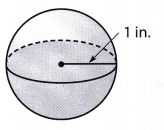Volume of the sphere = $$\frac{4}{3}$$πr3
V = $$\frac{4}{3}$$ ∙ 3.14 ∙ 13
V = 4.1867 in3
V ≈ 4.12 in3Diameter = 20 cm
Radius r = $$\frac{20}{2}$$ cm
Volume of sphere = $$\frac{4}{3}$$πr3
Volume = $$\frac{4}{3}$$ × 3.14 × 103
Volume = 4186.66 cm3
Volume ≈ 4186.7 cm3

Question 5.
A sphere has a radius of 1.5 feet. ___________
Volume of sphere = $$\frac{4}{3}$$πr3
Volume = $$\frac{4}{3}$$ × 3.14 × 1.53
Volume = 14.13 ft3
Volume ≈ 14.1 ft3

Question 6.
A sphere has a diameter of 2 yards. ___________
Diameter = 2 yards
Radius r = $$\frac{2}{2}$$ yards
Volume of sphere = $$\frac{4}{3}$$πr3
Volume = $$\frac{4}{3}$$ × 3.14 × 13
Volume = 4.1866 yd3
Volume ≈ 4.2 yd3

Question 7.
A baseball has a diameter of 2.9 inches. Find the volume of the baseball. Round your answer to the nearest tenth if necessary. Use 3.14 for π. (Example 2)
Diameter of baseball = 2.9 in
Radius r = $$\frac{2.9}{2}$$ in
Radius of baseball = 1.45 in
Volume of sphere = $$\frac{4}{3}$$πr3
Volume = $$\frac{4}{3}$$ × 3.14 × 1.453
Volume = 12.763 in3
Volume ≈ 12.8 in3

Question 8.
A basketball has a radius of 4.7 inches. What is its volume to the nearest cubic inch? Use 3.14 for π. (Example 2)
Volume of sphere = $$\frac{4}{3}$$πr3
Volume = $$\frac{4}{3}$$ × 3.14 × 4.73
Volume = 434.6723 in3
Volume ≈ 434.67 in3

Question 9.
A company is deciding whether to package a ball in a cubic box or a cylindrical box. In either case, the ball will touch the bottom, top, and sides.
(Explore Activity)a. What portion of the space inside the cylindrical box is empty? Explain.
The volume of the cylinder is
Vcylinder = πr2h
Since the ball touches the bottom, top, and sides, then the height of the cylinder will be equal to 2r.
Vcylinder = π ∙ r2 ∙ (2r)
Vcylinder = 2πr3
On the other hand, the voLume of the sphere is
Vsphere = $$\frac{4}{3}$$πr3
The volume of the empty space inside the cylindrical box is found by subtracting the volume of the sphere from the volume of the cylinder:
Vcylinder – Vsphere = 2πr3 – $$\frac{4}{3}$$πr3
= (2 – $$\frac{4}{3}$$)πr3
= ($$\frac{6}{3}-\frac{4}{3}$$)πr3
= $$\frac{2}{3}$$πr3

b. Find an expression for the volume of the cubic box.
The volume of a cube with side a is
Vcube = a3
Since the ball touches the bottom, top, and sides, then the side of the cube will be equal to 2r.
Vcube = (2r)3
Vcube = 8r3

c. About what portion of the space inside the cubic box is empty? Explain.
The volume of the empty space inside the cubical box is found by subtracting the volume of the sphere from the volume of the cube:
Vcube – Vsphere = 8r3 – $$\frac{4}{3}$$πr3
= (8 – $$\frac{4}{3}$$π)r3
= (8 – $$\frac{4}{3}$$ ∙ 3.14)r3
≈ (8 – 4.2)r3
≈ 3.8r3

Essential Question Check-In

Question 10.
Explain the steps you use to find the volume of a sphere.
Step 1: The radius of the sphere is found out
Step 2: The volume of the sphere is $$\frac{4}{3}$$πR3; where R stands for the radius.
Step 3: Put the value of radius in the equation of volume.
Step 4 : Calculate the volume.

Find the volume of each sphere. Round your answers to the nearest tenth if necessary. Use 3.14 for π.

Question 11.
The volume of the sphere with a radius of 3.1 meters is
V = $$\frac{4}{3}$$πr3
V = $$\frac{4}{3}$$ ∙ 3.14 ∙ (3.1)3
V ≈ 124.7 m3

diameter of 18 inches __________
The diameter of the sphere is 18 inches, which means that its radius is 9 inches. The volume of this sphere is
V = $$\frac{4}{3}$$πr3
V = $$\frac{4}{3}$$ ∙ 3.14 ∙ (9)3
V = 3052.08
V ≈ 3052.1 inches3

Question 13.
r = 6 in. _____________
The volume of the sphere with a radius of 6 inches is
V = $$\frac{4}{3}$$πr3
V = $$\frac{4}{3}$$ ∙ 3.14 ∙ (6)3
V = 904.32
V ≈ 904.3 inches3

Question 14.
d = 36 m _____________
Diameter d = 36 m
Radius r = $$\frac{36}{2}$$ = 18 m
V = $$\frac{4}{3}$$πr3
V = $$\frac{4}{3}$$ ∙ 3.14 ∙ (18)3
V = 24416.64 m3

Question 15.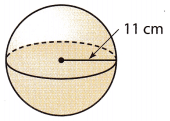The volume of the sphere with a radius of 11 centimeters is
V = $$\frac{4}{3}$$πr3
V = $$\frac{4}{3}$$ ∙ 3.14 ∙ (11)3
V ≈ 5572.5 cm3

Question 16.The diameter of the sphere is 2.5 feet, which means that its radius is 1.25 feet. The volume of this sphere is
V = $$\frac{4}{3}$$πr3
V = $$\frac{4}{3}$$ ∙ 3.14 ∙ (1.25)3
V ≈ 8.2 feet3

The eggs of birds and other animals come in many different shapes and sizes. Eggs often have a shape that is nearly spherical. When this is true, you can use the formula for a sphere to find their volume.

Question 17.
The green turtle lays eggs that are approximately spherical with an average diameter of 4.5 centimeters. Each turtle lays an average of 113 eggs at one time. Find the total volume of these eggs, to the nearest cubic centimeter.
The diameter of an egg (sphere) is 4.5 centimeters, which means that its radius is 2.25 centimeters. The volume of a single egg is
V = $$\frac{4}{3}$$πr3
V = $$\frac{4}{3}$$ ∙ 3.14 ∙ (2.25)3
V ≈ 47.68875 cm3
Therefore, the total volume of 113 eggs is
113 ∙ V = 113 ∙ 47.68875
= 5388.82875
≈ 5389 cm3
The total volume of 113 eggs is approximately 5388.8 cm3

Question 18.
Hummingbirds lay eggs that are nearly spherical and about 1 centimeter in diameter. Find the volume of an egg. Round your answer to the nearest tenth.
The diameter of the egg (sphere) is 1 centimeter, which means that its radius is 0.5 centimeters. The volume of a single egg is
V = $$\frac{4}{3}$$πr3
V = $$\frac{4}{3}$$ ∙ 3.14 ∙ (0.5)3
V ≈ 0.5233
V ≈ 0.5 cm3

Question 19.
Fossilized spherical eggs of dinosaurs called titanosaurid sauropods were found in Patagonia. These eggs were 15 centimeters in diameter. Find the volume of an egg. Round your answer to the nearest tenth.
Diameter of an egg (d) = 15 cm
Radius (r) = $$\frac{15}{2}$$ = 7.5 cm
Volume = $$\frac{4}{3}$$πr3
Volume = $$\frac{4}{3}$$ × 3.14 × 7.53
Volume = 1766.25 cm3
Volume ≈ 1766.3 cm3

Question 20.
Persevere in Problem Solving An ostrich egg has about the same volume as a sphere with a diameter of 5 inches. If the eggshell is about $$\frac{1}{12}$$ inch thick, find the volume of just the shell, not including the interior of the egg. Round your answer to the nearest tenth.
The diameter of the egg, including the eggshell, is
d = 5 + (2 ∙ $$\frac{1}{12}$$)
d = 5 + $$\frac{1}{6}$$
d = $$\frac{31}{6}$$ inches
Therefore, the radius of the egg, including the eggshell, is
r = $$\frac{d}{2}$$
r = $$\frac{31}{12}$$
r ≈ 2.583 inches
The volume of the egg, including the eggshell, is
V1 = $$\frac{4}{3}$$πr3
V1 = $$\frac{4}{3}$$ ∙ 3.14 ∙ (2.583)3
V1 ≈ 72.2 inches3
The diameter of the egg, excluding the eggshell, is 5 inches, which means that its radius ¡s 25 inches The volume of the egg, excluding the eggshell, is
V2 = $$\frac{4}{3}$$πr3
V2 = $$\frac{4}{3}$$ ∙ 3.14 ∙ (2.5)3
V2 ≈ 65.4 inches3
To find the volume of just the shell, we subtract the volume of the egg excluding the shell from the volume of the egg including the shell.
V1 – V2 = 72.2 – 65.4
V1 – V2 = 6.8 inches3
The volume of just the eggshell is approximately 6.8 inches3.

Go Math Book Grade 8 Answer Key Practice and Homework Lesson 9.3 Question 21.
Multistep Write the steps you would use to find a formula for the volume of the figure at right. Then write the formula.Height of cylinder = r
Step 1: Find the formula for the volume of the hemisphere.
Volume of hemisphere = $$\frac{\frac{4}{3} \pi r^{3}}{2}=\frac{2}{3} \pi r^{3}$$

Step 2: Find the formula for the volume of a cylinder.
Volume of cylinder = πr2h
Height of cylinder is equal to the radius, so
Volume of cylinder = πr3

Step 3: Add both the volume expressions:
Total volume = $$\frac{2}{3}$$πr3 + πr3 = $$\frac{5}{3}$$πr3

Question 22.
Critical Thinking Explain what happens to the volume of a sphere if you double the radius.
Volume V1 = $$\frac{4}{3}$$πr3
Volume V2 = $$\frac{4}{3}$$π(2r)3
= 8 × $$\frac{4}{3}$$πr3
= 8V1
= 8 (initial volume)
By doubling the radius of the sphere we make the volume 8 times the initial value.

Question 23.
Multistep A cylindrical can of tennis balls holds a stack of three balls so that they touch the can at the top, bottom, and sides. The radius of each ball is 1.25 inches. Find the volume inside the can that is not taken up by the three tennis balls.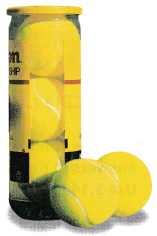Radius of a tennis ball = 1.25 in
Diameter of one ball = 2 × 1.25 = 2.5 in
Height of the cylinder = 2.5 × 3 = 7.5 in
Radius of base of cylinder = 125 in
Volume of cylinder (V1)= πr2h
V1 = 3.14 × 1.252 × 7.5
V1 = 36.796875 in3
V1 ≈ 36.8 in3
Volume of a ball (all three) (V2) 3 × $$\frac{4}{3}$$πr3
V2 = 4 × 3.14 × 1.253
V2 = 24.53125 in3
V2 ≈ 24.5 in3
Volume of empty space = Volume of cylinder – Volume of a ball (all three)
= 36.8 – 24.5 = 12.3 in3

H.O.T. Focus on Higher Order Thinking

Question 24.
Critique Reasoning A sphere has a radius of 4 inches, and a cube-shaped box has an edge length of 7.5 inches. J.D. says the box has a greater volume, so the sphere will fit in the box. Is he correct? Explain.
Volume of sphere = $$\frac{4}{3}$$πr3 = $$\frac{4}{3}$$π43 = 267.9467 in3
Volume of sphere ≈ 267.9 in3
Volume of cube-shaped box = 7.5 3 = 421.875 in3
Volume of cube-shaped box ≈ 421.9 in3
Volume of cube > Volume of a sphere
But the base of the cube has an area of (7.5 × 7.5) = 56.25 in2 while the cross-action area of the sphere is:
πr2 = 3.14 × 42 = 50.24 in2
The cross-section area of the sphere is less than that of a cube. J.D. is right and the ball (sphere) will fit in the cube.

Question 25.
Critical Thinking Which would hold the most water: a bowl in the shape of a hemisphere with radius r, a cylindrical glass with radius r and height r, or a cone-shaped drinking cup with radius r and height r? Explain.
The volume of a sphere with a radius r is
Vsphere = $$\frac{4}{3}$$πr3
Therefore, the volume of a hemisphere is
Vhemisphere = $$\frac{V_{\text {sphere }}}{2}$$
Vhemisphere = $$\frac{2}{3}$$πr3
The volume of a cylinder with radius r and height r is
Vcylinder = πr2h
Vcylinder = πr3
The volume of a cone with radius r and height r is
Vcone = $$\frac{1}{3}$$πr2h
Vcone = $$\frac{1}{3}$$πr3
As we can notice
Vcone < Vhemisphere < Vcylinder
Therefore, the cylindrical glass with radius r and height r will hold the most water.

Question 26.
Analyze Relationships Hari has models of a sphere, a cylinder, and a cone. The sphere’s diameter and the cylinder’s height are the same, 2r. The cylinder has radius r. The cone has diameter 2r and height 2r. Compare the volumes of the cone and the sphere to the volume of the cylinder.
Diameter of sphere = 2r
Radius r = $$\frac{d}{2}$$ = $$\frac{2r}{2}$$ = r
Volume of sphere = $$\frac{4}{3}$$πr3
Height of cylinder 2r
Volume of cylinder = πr2h = πr2(2r) = 2πr3
Diameter of cone = 2r
Radius of cone r = $$\frac{d}{2}$$ = $$\frac{2r}{2}$$ = r
Height of cone 2r
Volume of cone = $$\frac{1}{3}$$πr2h = $$\frac{2}{3}$$πr2r = $$\frac{2}{3}$$πr3
Volume of cylinder > Volume of sphere > Volume of cone
2πr3 > $$\frac{4}{3}$$πr3 > $$\frac{2}{3}$$πr3

Question 27.
A spherical helium balloon that is 8 feet in diameter can lift about 17 pounds. What does the diameter of a balloon need to be to lift a person who weighs 136 pounds? Explain.
Radius = r = $$\frac{8}{2}$$ = 4 ft
Volume of a spherical helium balloon = $$\frac{4}{3}$$πr3 = 43($$\frac{4}{3}$$π)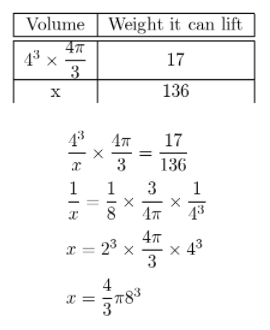Volume of balloon which can lift 136 pounds is equal to $$\frac{4}{3}$$π83.